# Electronics Tutorial

Welcome to the electronics tutorial! If you want to learn electronics, it's a great place to start. You can also revise for your GCSE here, as it's written to GCSE standard for the year 2000 (so if you're taking it in 1999, forget it. but have a look anyway!). It's suitable for all, and anyone can learn electronics using a combination of this & the other material available on my site. Whether you want to learn as a hobby, are taking a course in it, or just want to brush up on a few points, there should be something here to help you.
If you have any questions about electronics, this tutorial, or just fancy a chat, you can e-mail me on alex_tb@hotmail.com . If you've got a PhD in electronics or something, & you think I've stated something wrongly in here, please e-mail me & tell me about it, so I can correct it.

### 1. Electronic systems

All circuits can be designed as a system.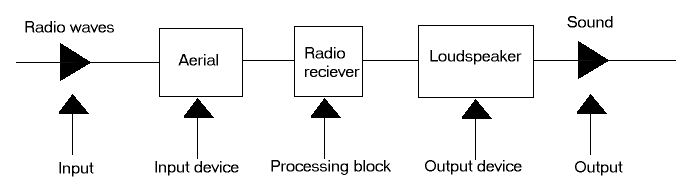The processing can the be further subdivided: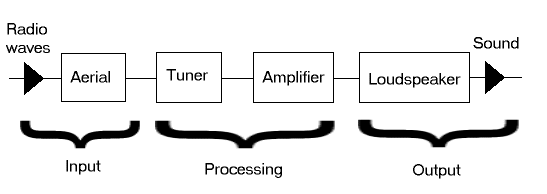Each block can then be designed in isolation, and the blocks coupled together. We need to know the input & output properties of each block, but no details of how they work.

NB. Each block also needs a power supply input, but this is not normally shown on the diagram.

### 2. The resistor

A resistor has a resistance, which is measured in ohms. If you haven't got the symbol font installed, the following character will not display correctly - W . This is the symbol for Ohms.

Wire wound resistor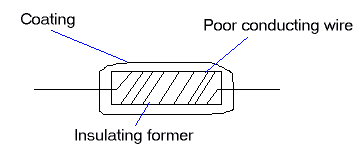• For a higher resistance, we use a longer or thinner wire. This is quite an accurate method.
• They are temperature-stable.
• They can take a large power (gets hot) without failing.
• But they are expensive.• Higher resistance - thinner carbon or narrower tracks increases the resistance.

Resistors are made in large batches, and very cheaply. This means that the resistance value obtained is rarely exactly right. This variation is known as the production spread. The accuracy of a resistor is given to ± a percentage. The ± means that it is within + or - that amount. The accuracy is known as its tolerance.

Colour coding

 Black 0 Brown 1 Red 2 Orange 3 Yellow 4 Green 5 Blue 6 Violet 7 Grey 8 White 9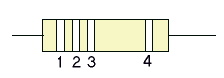Band 1 = First digit of resistance
Band 2 = Second digit of resistance
Band 3 = Number of following zeros
Band 4 = Tolerance

Tolerances

No band = ± 20 %
Silver = ± 10 %
Gold = ± 5 %

The resistor printed code

R = ohms
K = thousands of ohms
M = millions of ohms

The position of the letter determines the decimal point.

1800 = 1K8
120 = 120R

Tolerances are shown by using postfixes:

J = ± 5 %
K = ± 10 %
M = ± 20%

So:

56000 ± 10 % = 56K0K
6800000 ± 5 % = 6M8J

3. Current

Voltage = Current x Resistance
V = I x R
Volts = amps x ohms
Volts = milliamps x kilo ohms

NB. 1mA = 0.001 A
1 Kilo ohm = 1000 ohms

4. Resistances in seriesR1 R2 V1 (V) V2 (V) V3 (V) VT (V2+V3) 2K2 3K3 15.12 6.50 8.60 15.10 4K7 1K2 15.12 12.08 3.04 15.12 270R 150R 15.12 9.25 5.84 15.09 39K 69K 15.12 5.46 9.61 15.07

From this experiment, we can conclude: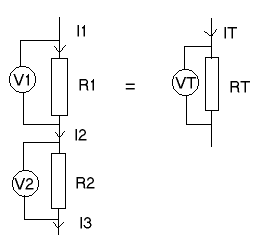• The currents are all equal ( IT = I1 = I2 = I3 )
• The voltages (Potential differences) add together ( VT = V1 + V2 )
• The resistances add together ( RT = R1 + R2)

5. Parallel circuits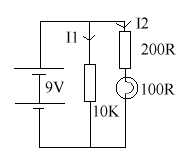The 2 circuits on parallel each have 9V across them.

I1 = V / R = 9 / 10 = 0.9 mA

I2 = V / R = 9 / 0.3 = 30 mA

So the total current from this battery is 30.9 mA. Note that the resistance for the second half of the circuit = 300R = 0K3 , because 200 + 100 =300 .

Next page